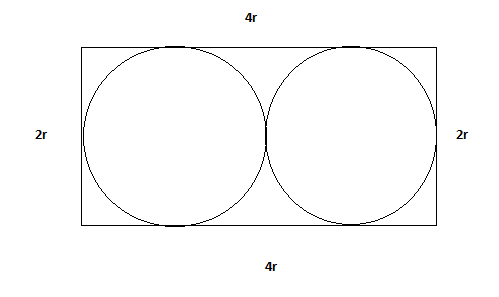Question 9

# A jogging park has two identical circular tracks touching each other, and a rectangular track enclosing the two circles. The edges of the rectangles are tangential to the circles. Two friends, A and B, start jogging simultaneously from the point where one of the circular tracks touches the smaller side of the rectangular track. A jogs along the rectangular track, while B jogs along the two circular tracks in a figure of eight. Approximately, how much faster than A does B have to run, so that they take the same time to return to their starting point?

SolutionLet the radius of 2 circles be r . Speed of A would be 12r/t and Speed of B would be 4*Pi*r/t . To find percentage faster B have to run than A we have : (4*Pi - 12)*100/ (4*Pi) = 4.46% approx. Hence, option D.